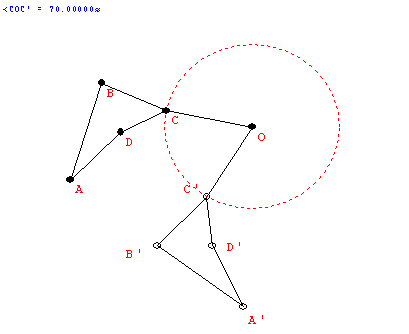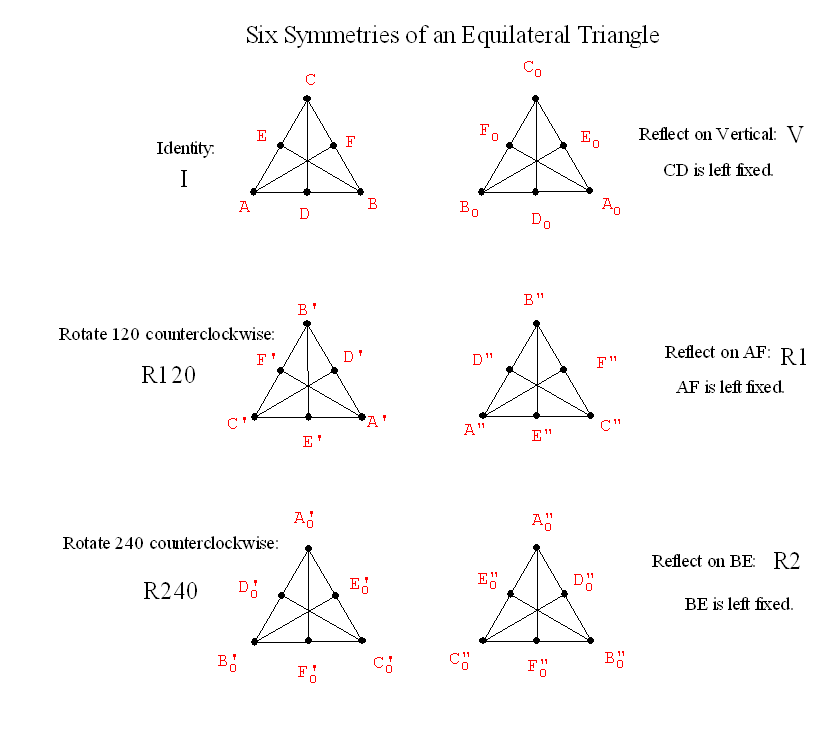September 16
Discuss Project proposal... Guidelines are now on the web! Form partnerships?

Review from last class:

Number of polygons
around a vertex
Equation for angle sum = 360 Equivalent Arithmetic equation Solutions to the arithmetic equations.
3: n , k, p 180 - 360/n+180 - 360/k+180 - 360/p = 360 1/n+1/k+1/p =1/2  6 6 6 5 5 10 4 5 20 4 6 12 4 8 8 3 7 42 3 8 24 3 9 18 3 10 15 3 12 12

4:  n, k, p, z 180 - 360/n+180 - 360/k+180 - 360/p 180 - 360/z = 360 1/n+1/k+1/p +1/z =2/2 =1  4 4 4 4 3 3 4 12 3 3 6 6 3 4 4 6

5:  n, k, p, z, w 180 - 360/n+180 - 360/k+180 - 360/p+180 - 360/z+180 - 360/w = 360 1/n+1/k+1/p +1/z+1/w =3/2  3 3 3 3 6 3 3 3 4 4

• Local consideration about a polygon:
• Examples: If there is an equilateral triangle involved with 2 other polygons, then the other two ploygons must have the same number of sides. Because: if there were two different polygons aroung the triangle, then on vertex would not have all three polygons sharing that vertex. (such as 3-10-15)
• Similar considerations can eliminate tilings using a single pentagon and two other distinct tiles. (such as 4-5-20).

• Semiregular Tilings: global results!
Look at the results using wingeometry.
• Student lesson (Math Forum) a place for further explorations on-line.
• Show FAPP video on Patterns
• as review of tilings so far and
• Introduces Penrose Tiles  K&M section 4.3 as source for possible protfolio/project ideas.
Duality Activity
Why is this called "duality"?
Back and forth in the construction
We place a point in each  region(polygon) .
Every vertex of the old figure is surrounded by a region( polygon) in the new figure.
"Having two forms"...   point.... region

Other dualities?
 right rich light good life up hot short right sweet denotation salt sun male yin left poor dark evil death down cold tall wrong sour connotation pepper moon female yang

Symmetry Ideas
• Reflective symmetry: BI LATERAL SYmMETRY
• Folding line: "axis of symmetry"
• The "flip."
• The "mirror."
•• R(P) = P'  : A Transformation. Before: P .... After : P'

• If P is on the line (axis), then F(P)=P. "P remains fixed by the reflection."
• If P is not on the axis, then the line PP' is perpendicular to the axis and if Q is the point of intersection of PP' with the axis then m(PQ) = m(p'Q).

• Definition: We say F has a reflective symmetry wrt a line l if  there is a reflection  R about the line l where  R(P) is still an element of F for every P in F....i.e.. R (F) = F.
• Examples of reflective symmetry:

• Rotational Symmetry.
• Center of rotation. "rotational pole" (usually O) and angle/direction of rotation.
• The "spin."
•• R(P) = P' : A transformation
• If O is the center then F(O) = O.
• If the angle is 360 then F(P) = P for all P.... called the identity transformation.
• If  the angle is between 0 and 360 then only the center remains fixed.
• For any point P the angle POP'  is the same.
• Examples of rotational symmetry.

•  Now what about finding all the reflective and rotational symmetries of a single figure?

•• Why are there only six?
• What about combining transformations to give new symmetries: